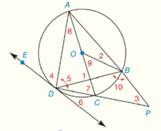Chapter 6.CR, Problem 30CRElementary Geometry For College St...

7th Edition
Alexander + 2 others
ISBN: 9781337614085

Solutions

Chapter
SectionElementary Geometry For College St...

7th Edition
Alexander + 2 others
ISBN: 9781337614085
Textbook Problem

G i v e n :                       ⊙ O   w i t h   d i a m e t e r   A C -   a n d   t a n g e n t   D E ⃡                                                                       m A D ^ = 136 °   a n d   m B C ^ = 50 °                 F i n d :                           T h e   m e a s u r e s   o f   ∠ 1   t h r o u g h   ∠ 10To determine

To Find: The measures of 1 through 10, if O with diameter AC- and tangent DE. mAD^=136° and mBC^=50°.Explanation

Concept:

Definition:

A central angle of a circle is an angle whose vertex is the center of the circle and whose sides are radii of the circle.

Definition:

An inscribed angle of a circle is an angle whose vertex is a point on the circle and whose sides are chords of the circle.

Theorem 6.1.2:

The measure of an inscribed angle of a circle is one-half the measure of its intercepted arc.

Theorem 6.1.8:

Congruent chords are located at the same distance from the center of a circle.

Theorem 6.1.9:

Angle inscribed in a semicircle is a right angle.

Theorem 6.2.2:

The measures of an angle formed by two chords that intersect within a circle is one-half the sum of the measures of the arcs intercepted by the angle and its vertical angle.

Theorem 6.2.4:

The measure of an angle formed by a tangent and a chord drawn to the point of tangency is one-half the measure of the intercepted arc.

Theorem 6.2.5:

The measure of an angle formed when two secants at a point outside the circle is one-half the difference of the measures of the two intercepted arcs.

Calculation:

Given O with diameter AC- and tangent DE. mAD^=136° and mBC^=50°.

Since mBC^=50°, its central angle is 9=50°.

By Theorem 6.1.9:

Angle inscribed in a semicircle is a right angle.

So, 5=90°

By Theorem 6.2.4:

The measure of an angle formed by a tangent and a chord drawn to the point of tangency is one-half the measure of the intercepted arc.

So, 6=12mDC^ and 4=12AOD=12×136°=68°

Also, 7=12AOD=12×136°=68°

Since sum of the three angles in ADC=180°

5+7+8=180°.

90+68+8=180°.

158°+8=180°.

8=180°-158°

8=22°

And 6=12mDC^=442=22°

By Theorem 6.2.2:

The measures of an angle formed by two chords that intersect within a circle is one-half the sum of the measures of the arcs intercepted by the angle and its vertical angle

Still sussing out bartleby?

Check out a sample textbook solution.

See a sample solution

The Solution to Your Study Problems

Bartleby provides explanations to thousands of textbook problems written by our experts, many with advanced degrees!

Get Started

Convert the expressions in Exercises 6584 to power form. x23

Finite Mathematics and Applied Calculus (MindTap Course List)

Simplify each of the following so that only positive exponents remain.

Mathematical Applications for the Management, Life, and Social Sciences

In Exercises 63-68, rationalize the denominator. 66. 5x23x

Applied Calculus for the Managerial, Life, and Social Sciences: A Brief Approach

Find tan if sin=13 and terminates in QI.

Trigonometry (MindTap Course List)

The graph at the right has a local maximum at x ______. a) 1, 3, and 5 b) 2 c) 4 d) 2 and 4

Study Guide for Stewart's Single Variable Calculus: Early Transcendentals, 8th

True or False: is monotonic.

Study Guide for Stewart's Multivariable Calculus, 8th